Home

 << Previous      2  3  4  5  ...24    Next >>

Four-dimensional Sacred Geometries

 It was shown in Sacred geometry/Platonic solids and in Superstrings as sacred geometry/Platonic solids that the five regular polyhedra represent stages in the unfolding in 3-dimensional space of the archetypal, mathematical structure of the 2-dimensional, inner form of the Tree of Life. That analysis raises the question: does sacred geometry exist as well amongst some of the objects in 4-dimensional space that professional mathematicians know inhabit it? Unable to accept the notion of "sacred" vis-à-vis geometry whatever its dimensionality (let alone incapable of recognising examples of it), most mathematicians would, no doubt, answer this question in the negative unless they happened to be Platonists. This section will analyze the six 4-dimensional counterparts of the Platonic solids (the so-called "polychorons") without such bias in order to uncover evidence for their possession of sacred geometry. There is good reason for focusing upon a space with four dimensions. This particular dimensionality is unique in permitting the maximum number of convex, regular polytopes because the number reduces to (and stays constant at) three for all spaces with more than four dimensions. Readers who want to study in more detail the material to be presented here should read Article 62. In the meantime, a characteristic of 3-dimensional, sacred geometries discussed in Article 53 will be shown in the next page to belong also to the 421 polytope, the 8-dimensional polytope whose 240 vertices determine the root vectors of E8, the rank-8, simple, exceptional Lie group discovered in 1984 to be at the heart of superstring theory. The 4-dimensional projection of its 240 vertices are the vertices of two concentric, but different sized, 600-cells, which turn out to embody structural parameters (namely, 840, 1680 & 16800) of the basic particle remote viewed over a century ago by the Theosophists Annie Besant & C.W. Leadbeater. Because it is highly implausible to dismiss these features as due to chance, the discovery that the 421 polytope and various polychorons embody numbers that were obtained by remote-viewing has profound, scientific and religious consequences that reach far beyond pure mathematics and particle physics. It is added here because of its importance as hard, mathematical evidence for the existence of superstring constituent of quarks, as well as for their 10-fold structure. This is a property that is also embodied in various, 3-dimensional sacred geometries that have been found elsewhere on this website to represent in analogous ways the group mathematics of SO(32) & E8×E8, which are the two gauge symmetry groups governing superstring forces that are free of quantum anomalies. What is presented in this section is "smoking gun" proof that the units of matter that Besant & Leadbeater claimed to remote view are particular states of E8×E8 heterotic superstrings. Evidence that confirms this conclusion with its revolutionary implications will be provided in the pages of later sections.

1. The 6 polychorons

Definitions
A polytope is a geometric object with straight sides that exists in any number of dimensions. In two dimensions a polytope is a polygon and in three dimensions it is a polyhedron. If a polytope contains all the points on a segment joining any pair of points in it, the polytope is convex. An n-polytope is an n-dimensional polytope. An
n-polytope is regular if any set consisting of a vertex, an edge containing it, a 2-dimensional face containing the edge, and so on up to n−1 dimensions, can be mapped to any other such set by a symmetry of the polytope. The regular polygons form an infinite class of 2-polytopes. There are five regular, convex polyhedra, or 3-polytopes, known as the Platonic solids. There exist six types of regular, convex 4-polytopes known as the polychorons, but only three types of n-polytopes when n5.

The elements of a polytope are its vertices (0-polytopes, or so-called "0-faces"), edges (1-polytopes, or 1-faces), faces (2-polytopes, or 2-faces), cells (3-polytopes, or 3-faces) and so on. These elements can be represented by the following Schäfli symbols:

regular p-gon: {p}.

regular polyhedron with q regular p-gon faces meeting at a vertex: {p,q}.

regular 4-polytope with r {p,q} regular polyhedral cells around each edge: {p,q,r}.

and so on. The dual of the {p,q} polyhedron is the {q,p} polyhedron, i.e., vertices and faces are interchanged. It is self-dual when p = q. The dual of the 4-polytope {p,q,r} has the Schäfli symbol {r,q,p}. A 4-polytope is self-dual if it is identical to its dual. This means that a self-dual 4-polytope has the Schäfli symbol {p,q,p}. The Schäfli symbols of the five Platonic solids are:

tetrahedron: {3,3}.

octahedron: {3,4}.

cube: {4,3}.

icosahedron: {3,5}.

dodecahedron: {5,3}.

If a vertex of a polyhedron is sliced off, the surface created is bounded by a polygon called the vertex figure. A regular polyhedron has always a regular polygon as its vertex figure. A {p,q} regular polyhedron has the regular vertex figure {q}. The vertex figure of a polychoron is a polyhedron, seen by the arrangement of neighbouring vertices around a given vertex. For regular polychora with the Schäfli symbol {p,q,r}, this vertex figure is a {q,r} regular polyhedron. The vertex figure for an n-polytope is an (n1)-polytope. An edge figure for a polychoron is a polygon, seen by the arrangement of faces around an edge. For regular {p,q,r} polychora, this edge figure will always be a regular polygon {r}. Regular polychora with Schäfli symbol {p,q,r} have r polyhedral cells of type {p,q} around each edge, faces of type {p}, edge figures {r} and vertex figures {q,r}.

Table 1a lists the geometrical elements making up the six convex, regular polychorons and Table 1b lists the number & types of polytopes meeting at each vertex, edge, face & cell:

Table 1a. The geometrical composition of the regular polychorons.

Table 1b. Number of vertices, edges, faces & cells contained by/meeting at each vertex, edge, face & cell.

 Polychoron Schäfli symbol {p,q,r} Vertices V {q,r} Edges E {r} Faces F {p} Cells C {p,q} Dual 5-cell {3,3,3} 5 {3,3} 10 {3} 10 {3} 5 {3,3} Self 8-cell {4,3,3} 16 {3,3} 32 {3} 24 {4} 8 {4,3} 16-cell 16-cell {3,3,4} 8 {3,4} 24 {4} 32 {3} 16 {3,3} 8-cell 24-cell {3,4,3} 24 {4,3} 96 {3} 96 {3} 24 {3,4} Self 120-cell {5,3,3} 600 {3,3} 1200 {3} 720 {5} 120 {5,3} 600-cell 600-cell {3,3,5} 120 {3,5} 720 {5} 1200 {3} 600 {3,3} 120-cell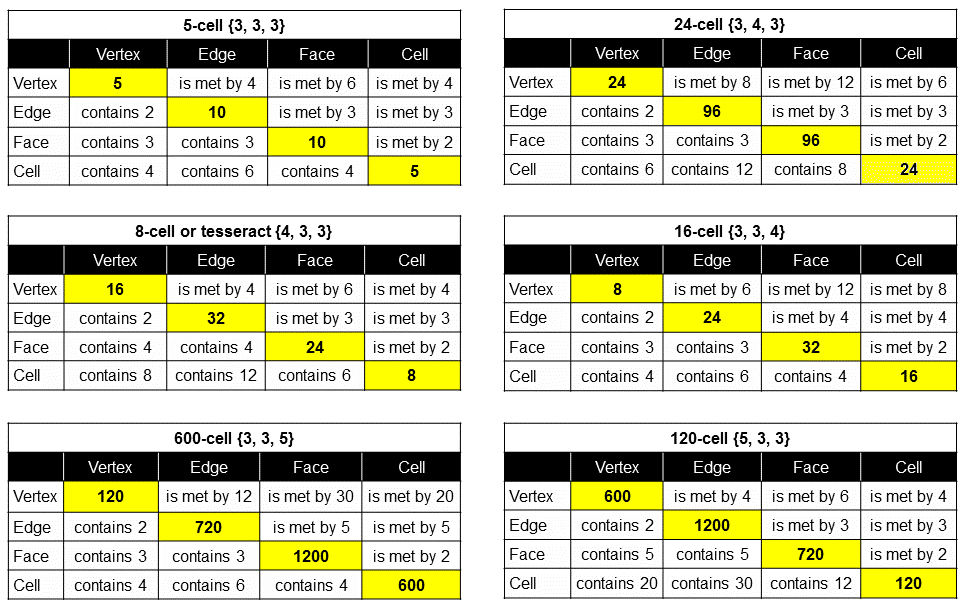(The Schäfli symbols of the vertex and edge figures are given for each vertex and edge count of a polychoron).

An n-cell is a polychoron with n polyhedral cells:

• The 5-cell (also called the 4-simplex, pentatope or pentachoron) with five vertices, 10 edges, 10 triangular faces & five tetrahedral cells is the 4-dimensional counterpart of the tetrahedron, both being self-dual;
• The 8-cell (also called the 4-cube, or tesseract) with 16 vertices, 32 edges, 24 square faces & eight cubic cells (four around each of its 32 edges) is the 4-dimensional counterpart of the cube;
• The 16-cell with eight vertices, 24 edges, 32 triangular faces & 16 tetrahedral cells (four meeting at each of 24 edges) is the 4-dimensional counterpart of the octahedron;
• The 24-cell, which was first discovered by mathematician Ludwig Schäfli in 1852, has no Platonic counterpart and — like the 5-cell — is self-dual. It has 24 vertices, 96 edges, 96 triangular faces & 24 octahedral cells, three being around each edge and six being around each vertex;
• The 120-cell with 600 vertices, 1200 edges, 720 pentagonal faces & 120 dodecahedral cells is the 4-dimensional counterpart of the dodecahedron with 12 pentagonal faces. It has three dodecahedra around each of its edges, just as the dodecahedron has three pentagonal faces meeting at each of its 20 vertices;
• The 600-cell with 120 vertices, 720 edges, 1200 triangular faces & 600 tetrahedral cells is the 4‑dimensional counterpart of the icosahedron with 20 triangular faces. It has five tetrahedra meeting at every one of its edges, just as the icosahedron has three triangles meeting at each of its 12 vertices.

Geometrical composition of the polychorons

We shall construct the faces and interiors of the polychorons from Type A triangles. A polychoron has V vertices, E edges, F faces & C cells, where

V − E + F − C = 0.

As regular m-gons (m = 3, 4 or 5), the faces of the polychoron are divided into their m sectors. Joining its vertices to its centre generates E internal triangles. Treating them as Type A triangles generates (E+1) new corners and (V+3E) new sides of 3E new triangles.

Faces

Number of corners of mF triangles in F faces ≡ C = V + F.

Number of sides of mF triangles ≡ S = E + mF.

Number of triangles ≡ T = mF.

Number of corners, sides & triangles ≡ N = C + S + T = V + E + (2m+1)F.

Interior

Number of corners of 3E triangles ≡ C′ = 1 + E

Number of sides of 3E triangles ≡ S′ = V + 3E.

Number of triangles ≡ T′ = 3E.

Number of corners, sides & triangles ≡ N′ = C′ + S + T′ = 1 + V + 7E.

Faces + interior

Total number of corners ≡ c = C + C′ = 1 + V + E + F.

Total number of sides ≡ s = S + S′ = V + 4E + mF.

Total number of triangles ≡ t = T + T′ = 3E + mF.

Total number of corners, sides & triangles ≡ n = N + N′ = 1 + 2V + 8E + (2m+1)F.

Table 2 lists the geometrical composition of the 6 polychorons:

Table 2. Geometrical composition of the faces and interiors of the 6 polychorons.

 Polychoron Faces Interior Total C S T N C′ S′ T′ N′ c s t n 5-cell 15 40 30 85 1+10 35 30 1+75 1+25=26 75 60 1+160 8-cell 40 128 96 264 1+32 112 96 1+240 1+72=73 240 192 1+504 16-cell 40 120 96 256 1+24 80 72 1+176 1+64=65 200 168 1+432 24-cell 120 384 288 792 1+96 312 288 1+696 1+216=217 696 576 1+1488 120-cell 1320 4800 3600 9720 1+1200 4200 3600 1+9000 1+2520=2521 9000 7200 1+18720 600-cell 1320 4320 3600 9240 1+720 2280 2160 1+5160 1+2040=2041 6600 5760 1+14400

(“1” denotes the centre of the polychoron).

Yod composition of the polychorons

Faces

Number of yods at corners of mF tetractyses ≡ C = V + F.

Number of hexagonal yods ≡ H = 2E + 3mF.

Total number of yods ≡ Y = C + H = V + 2E + (3m+1)F.

Number of yods on sides of tetractyses ≡ B = V + 2E + (2m+1)F.

Interior

Number of yods at corners of 3E tetractyses ≡ C′ = 1 + E.

Number of hexagonal yods ≡ H′ = 2V + 9E.

Total number of yods ≡ Y′ = C′ + H′ = 1 + 2V + 10E.

Number of yods on sides of tetractyses ≡ B′ = 2V + C′ + 6E = 1 + 2V + 7E.

Faces + interior

Number of yods at corners of (mF+3E) tetractyses ≡ c = C + C′ = 1 + V + E + F.

Number of hexagonal yods ≡ h = H + H′ = 2V + 11E + 3mF.

Total number of yods ≡ y = Y + Y′ = 1 + 3V + 12E + (3m+1)F.

Total number of boundary yods ≡ b = B + B′ = 1 + 3V + 9E + (2m+1)F.

Table 3 lists the yod composition of the 6 polychorons:

Table 3. Yod composition of the faces and interiors of the 6 polychorons.

 Polychoron Faces Interior Total C H B Y C′ H′ B′ Y′ c h b y 5-cell 15 110 95 125 1+10 100 1+80 1+110 1+25=26 210 1+175 1+235 8-cell 40 352 296 392 1+32 320 1+256 1+352 1+72=73 672 1+552 1+744 16-cell 40 336 280 376 1+24 232 1+184 1+256 1+64=65 568 1+464 1+632 24-cell 120 1056 888 1176 1+96 912 1+720 1+1008 1+216=217 1968 1+1608 1+2184 120-cell 1320 13200 10920 14520 1+1200 12000 1+9600 1+13200 1+2520=2521 25200 1+20520 1+27720 600-cell 1320 12240 9960 13560 1+720 6720 1+5280 1+7440 1+2040=2041 18960 1+15240 1+21000

(“1” denotes the centre of the polychoron).

5-cell
1) According to Table 1, its 5 vertices generate 10 edges of 10 triangular faces of 5 tetrahedra. i.e., it consists of 30 simplicial elements (31 elements, including itself), where

30 = 12 + 22 + 32 + 42.

The 5-cell embodies the number value of EL ("God"): AL = 1 + 30 = 31. This is the Godname of Chesed, the Sephirah below Chokmah, whose Godname YAH prescribes the 5 vertices & 10 edges of the 5-cell because it has the number value 15: YH = 5 + 10 = 15. This direct prescription by Godnames is unique to the tetrahedron and its 4-dimensional counterpart. This is shown by Table 4, which lists the first 11 simplexes and their simplicial composition:

Table 4. n-simplex elements.

 n-simplex 0-faces (vertices) 1-faces (edges) 2-faces 3-faces 4-faces 5-faces 6-faces 7-faces 8-faces 9-faces 10-faces Total = 2n+1−1 0-simplex  (point) 1 1 1-simplex  (line segment) 2 1 3 2-simplex  (triangle) 3 3 1 7 3-simplex  (tetrahedron) 4 6 4 1 15 4-simplex  (5-cell) 5 10 10 5 1 31 5-simplex 6 15 20 15 6 1 63 6-simplex 7 21 35 35 21 7 1 127 7-simplex 8 28 56 70 56 28 8 1 255 8-simplex 9 36 84 126 126 84 36 9 1 511 9-simplex 10 45 120 210 252 210 120 45 10 1 1023 10-simplex 11 55 165 330 462 462 330 165 55 11 1 2047

Although numbers in bold do appear in some of the cells, only the tetrahedron and the 5-cell have a total number of simplicial elements, including themselves, that is a Godname number. This indicates that they play a unique role in sacred geometries. One could, of course, have anticipated this in the case of the tetrahedron, which is the simplest Platonic solid and the building block of solid geometry. But it extends to its 4-dimensional counterpart. This is confirmed by the fact that, according to Table 2, the number value 15 of YAH (YH), the first half of Tetragrammaton (YHVH), the Godname of Chokmah expressing the creative power of God, is the number of points needed to construct the faces of the 5-cell from triangles. The value 5 of H is the number of vertices and the value 10 of Y is the additional number of corners of the 30 triangles in its faces. The number value 26 of YAHWEH, the full Godname of this Sephirah, is the total number of corners of the 60 triangles making up the 5-cell. The number value 21 of EHYEH, the Godname of Kether, is the number of corners other than vertices. The total number of their corners & sides is (26+75=101). This is the 26th prime number. 100 (=13+23+33+43) corners & sides surround the centre of the 5-cell.

2) The 30 triangles in the 10 faces comprise (15+40=55) corners & sides, where

 1 2 3 55 = 4 5 6 7 8 9 10

is the 10th triangular number. The faces comprise 85 geometrical elements, where

85 = 40 + 41 + 42 + 43,

of which 15 elements are points and 70 elements are lines & triangles. Compare these properties with the fact that the 2nd-order tetractys contains 85 yods, of which 15 yods are corners of the 10 1st-order tetractyses and 70 yods are hexagonal yods: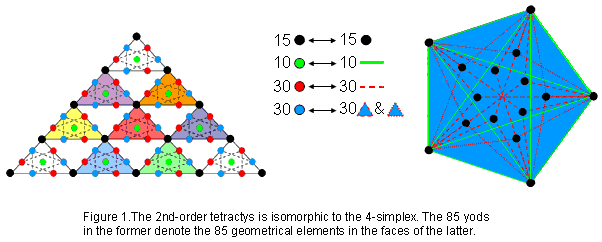The four rows of a 1st-order tetractys are traditionally thought to symbolise the sequence:

0-simplex1-simplex→2-simplex→3-simplex.

We now see that the 2nd-order tetractys symbolises the 4-simplex, the 15 black corners of the 10 1st-order tetractyses symbolizing the 15 points in 4-d space that are the corners of the 30 constituent triangles in its faces, the 10 green hexagonal yods at the centres of the 10 1st-order tetractyses denoting its 10 edges and the remaining 60 hexagonal yods (30 red, 30 blue) denoting the 30 triangular sectors of the 10 faces and their 30 remaining sides. Just as the tetrahedron (3-simplex) is the starting point in the build-up of the five regular polyhedra in 3-dimensional space, so the 4-simplex as the simplest polychoron commences the build-up of the six regular polychora in 4‑dimensional space. The Pythagorean representation of holistic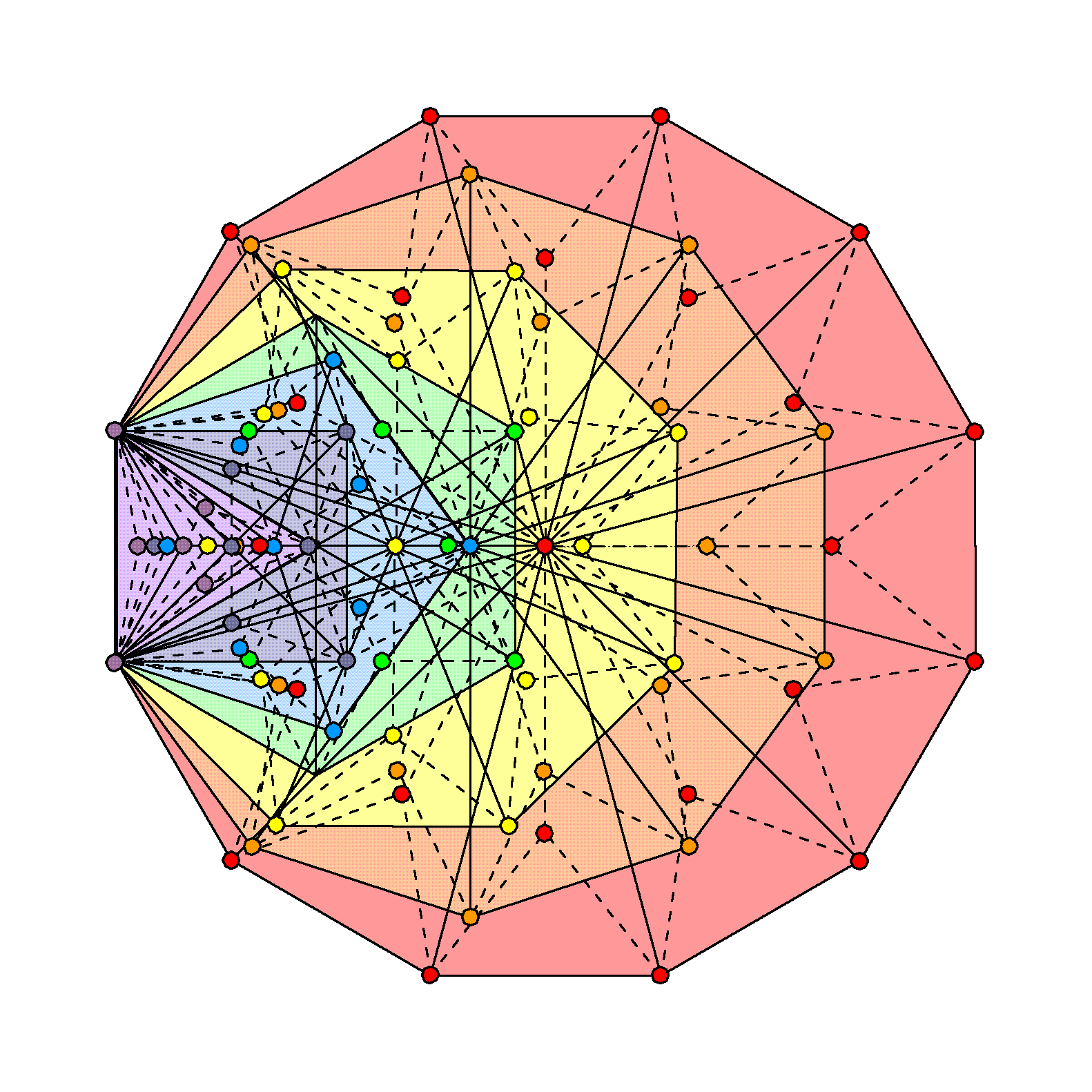Figure 2. The 141 triangular sectors of the 7 enfolded, Type B polygons have 85 corners unshared with the outer Tree of Life.

systems — the 2nd-order tetractys with 85 yods — represents the 4-simplex. 85 corners & triangles surround its centre. The counterpart of this in the inner Tree of Life are the 85 corners of the 141 triangles making up the 47 sectors of the seven enfolded Type B polygons that are intrinsic to them because none are corners of triangles belonging to the outer Tree of Life (Fig. 2). Notice that the diagram depicts no green coloured corners at the top, centre & bottom of the hexagon because these points coincide with Chokmah, Chesed & Netzach, which are located at corners of triangles in the outer Tree and so are shared with it. It might be thought that the seven enfolded polygons with 88 corners of their 141 triangles could, alternatively, embody the number 85 as the 85 corners outside the root edge that belong solely to each set of polygons enfolded in successive Trees of Life. However, this cannot be the ‘right’ embodiment because, in the former scheme, the embodiment of the superstring structural parameter 168 emerges naturally as the (84+84=168) corners of the 282 triangles composing the (47+47=94) sectors of the (7+7) enfolded polygons that are not corners of triangles in the outer Tree of Life, whereas in the latter scheme, the (7n+7n) polygons enfolded in n Trees of Life have 282n triangles with (170n+2) corners, but there is no reason for ignoring just one corner from each set of seven polygons so as to leave the required set of (168n+2) corners. The property that the (70+70) polygons enfolded in the 10‑tree should have 2820 triangles with 1680 corners (the inner Tree of Life representation of the 1680 turns of each helical whorl of the 10-dimensional UPA) leads, therefore, to a unique, geometrical interpretation of the number 85 as a holistic parameter. Its presence in the 4-simplex, shaping its faces, indicates that the Pythagorean archetypal symbol of the tetractys extends beyond 3-dimensional space into four dimensions through its next higher-order version.

3) (855=80) geometrical elements are needed to create the faces of the 5-simplex from five equidistant points in 4-dimensional space. This is the number of Yesod, the penultimate Sephirah. Its interior has 76 geometrical elements, where 76 is the number value of YAHWEH ELOHIM, the Godname of Tiphareth. 155 geometrical elements other than vertices surround its centre, with 50 points & lines in its faces. This shows how ELOHIM, the Godname of Binah with number value 50, and ADONAI MELEKH, the Godname of Malkuth with number value 155, prescribes the geometrical composition of the 5-simplex. It includes 65 lines other than edges, where 65 is the number value of ADONAI.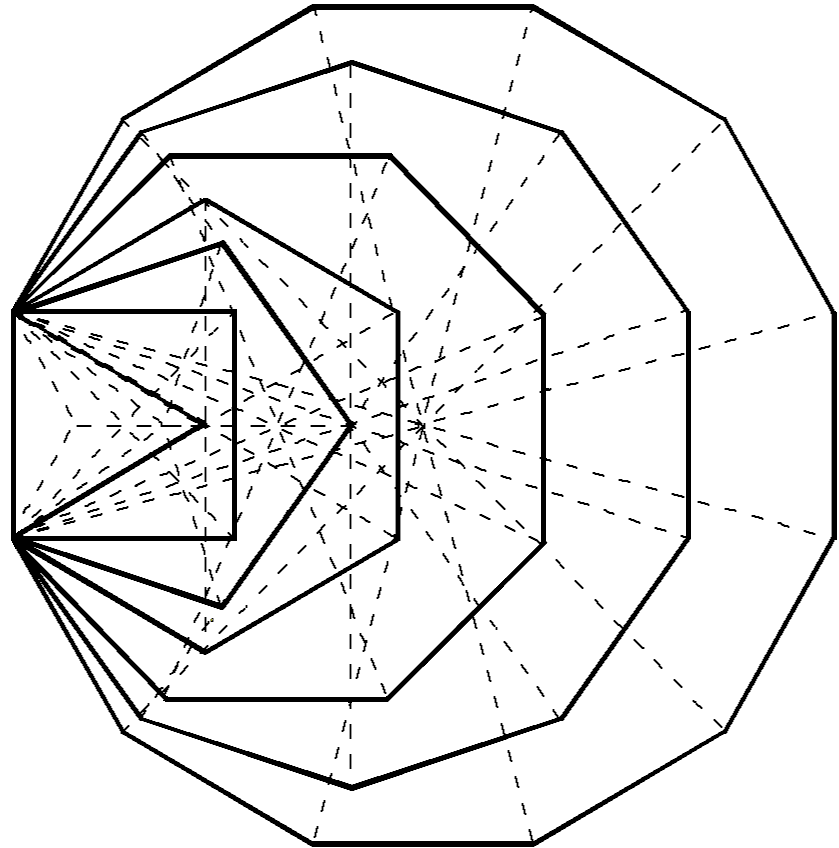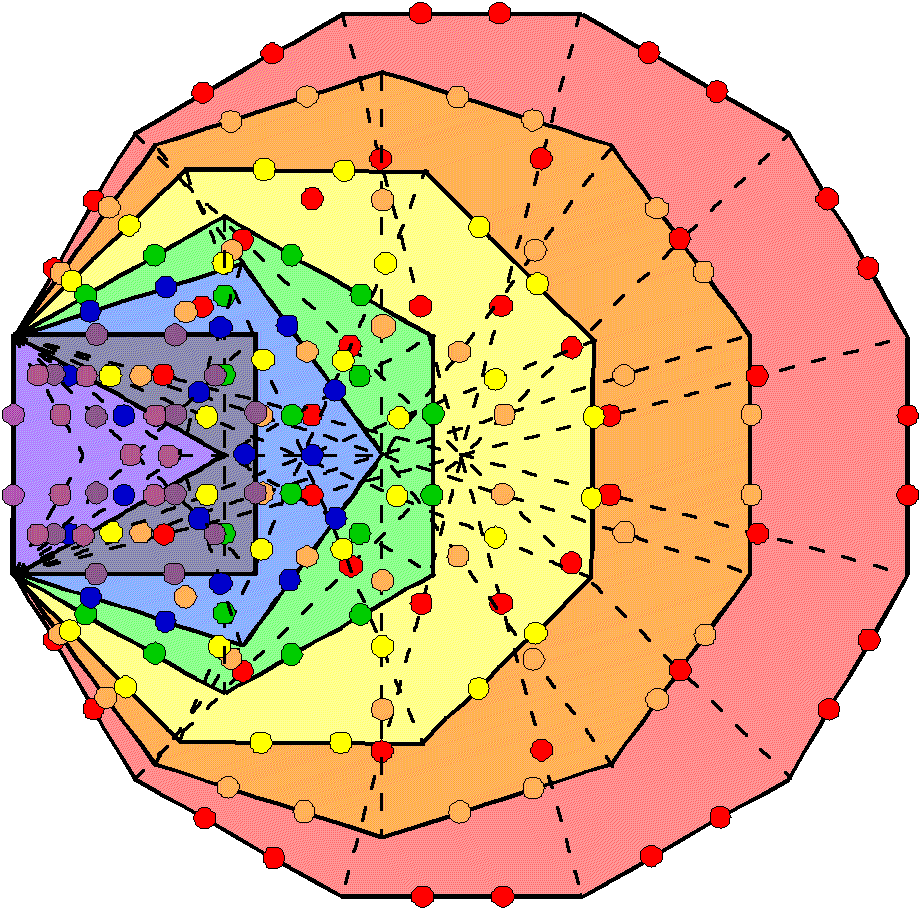Figure 3. 236 yods line the boundaries of the (7+7) enfolded polygons. The 7 enfolded polygons are composed of 176 corners, sides & triangles. 176 hexagonal yods line the 88 sides of the 47 tetractyses in the 7 enfolded polygons. Figure 4. The number 176 is a structural parameter of the inner Tree of Life.

4) In remarkable confirmation of the evidence presented earlier for the holistic nature of the 5-cell, the table indicates that it contains 236 yods. This is the number of yods on the boundary of the (7+7) enfolded polygons (Fig. 3). 70 yods inside the triangular faces line tetractyses. This is the number of corners of the (7+7) enfolded polygons that belong to the set of 236 boundary yods. The yod population of the 5-cell is the number of yods shaping the inner Tree of Life. Moreover, 176 yods line its 60 tetractyses. This is both the number of geometrical elements in the 47 sectors of the seven enfolded polygons and the number of hexagonal yods on the 88 sides of the 47 tetractyses (Fig. 4). In the former, the sum “1 + 175” appears as the topmost corner of the hexagon that coincides with the lowest corner of the hexagon enfolded in the next higher Tree and as the 175 geometrical elements intrinsic to the seven enfolded polygons. In the latter, the sum appears as one of the two hexagonal yods on the root edge that can be associated with one set of seven polygons and as the 175 hexagonal yods lining their 47 tetractyses. The possession of Tree of Life parameters by the 5-cell is strong evidence of its holistic nature as the simplest polychoron — the 4-dimensional version of the 4-vertex tetrahedron.

5) The 5-cell contains 210 (=21×10) hexagonal yods, where 21 is the number value of EHYEH, the Godname of Kether. It has 15 corners in its 10 faces and 11 corners in its interior, totalling 26, where 15 is the number of YAH and 26 is the number of YAHWEH. The 11:15 division of this Godname number is characteristic of sacred geometries and reflects the fact that YAH (YH) is a Godname in its own right: YHVH = (10+5) + (6+5) = 15 + 11.

The 5-cell

8-cell
1) The 96 triangular sectors of its 24 square faces are formed by 40 points and 128 straight lines, i.e., 168 points & lines. This is how the tesseract embodies the superstring structural parameter 168. 264 geometrical elements make up its faces. This is the number of yods in the seven enfolded polygons that constitute each half of the inner Tree of Life (Fig. 5). That the 8-cell conforms to the archetypal pattern of the inner Tree of Life and possesses other holistic properties, such as the 384 symmetries of the hyperoctahedral symmetry group BC4, is confirmed here.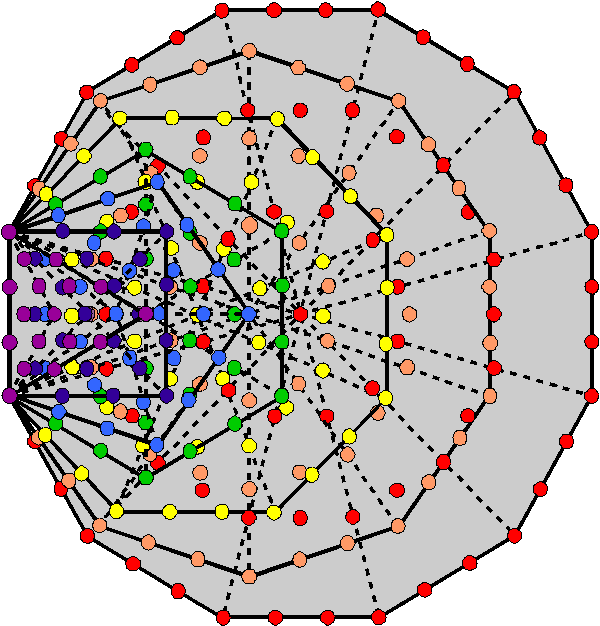Figure 5. The 47 sectors of the 7 enfolded polygons of the inner Tree of Life have 264 yods when these sectors are tetractyses.

2) 240 geometrical elements inside the 8-cell surround its centre. They comprise 128 corners & triangles and 112 sides. The 240 sides comprise 128 sides in its faces and 112 sides in its interior.

3) The 192 triangles in the 8-cell have 73 corners, where 73 is the number value of Chokmah.

4) There are 72 vertices, edges & square faces, where 72 is the number of Chesed. Including the eight cubic cells, the 8-cell has 80 vertices, edges, faces & cells, where 80 is the number of Yesod.

5) Its 192 tetractyses have 73 corners.

6) 296 yods line the 96 tetractyses in its 24 faces. 16 of these are vertices, leaving 280 yods needed to create these faces, where 280 is the number value of Sandalphon, the Archangel of Malkuth.

7) The 8-cell contains 672 hexagonal yods. This is the number of yods in the first four Platonic solids when their faces and interiors are constructed from tetractyses (see here).

The 8-cell

16-cell

1) Its 32 triangular faces contain 256 geometrical elements, where 256 = 44, showing how the Tetrad expresses its geometrical composition. The 16-cell has 168 triangles with 65 corners, where 65 is the number of ADONAI, the Godname of Malkuth, and 168 is the number of Cholem Yesodoth, the Mundane Chakra of this Sephirah. 432 geometrical elements surround its centre, demonstrating the role of the integers 1, 2, 3 & 4 symbolised by the tetractys in expressing properties of regular polyhedra and polychorons.

2) There are 80 vertices, edges, triangular faces & tetrahedral cells. where 80 is the number of Yesod.

3) 280 yods line the 96 tetractyses in its faces. This is a remarkable, chance-defying conjunction in the same geometrical context of the numbers of the Godname (65), Archangel (280) & Mundane Chakra (168) of the same Sephirah (Malkuth). This number reappears because the 16-cell has eight fewer vertices than the 8-cell but eight more faces, whilst it has eight fewer edges, so that it has (8×2=16) fewer yods in its faces, i.e., the same number of yods as in the 8-cube other than its 16 vertices.

4) 64 (=43) corners surround its centre, where 64 is the number value of Nogah, the Mundane Chakra of Netzach.

5) Its 32 faces have 336 hexagonal yods. This number is discussed widely in this website as a superstring structural parameter embodied in sacred geometries, being the number of turns in one revolution of each helical whorl of a UPA (subquark state of the E8×E8 heterotic superstring) around its axis of spin.

The 16-cell

24-cell

1) The 96 triangular faces consist of 288 triangles with 120 corners and 384 sides, where 120 is the sum of the first 10 odd integers after 1 and 288 = 11 + 22 + 33 + 44 = 1!×2!×3!×4!. It has 288 internal triangles, so that 576 triangles are present, where 576 = 242 = 12×22×32×42. Compare these properties with the following facts about the inner Tree of Life:

• 288 yods line the boundaries of the (7+7) separate, Type A polygons;
• 288 yods surround the centres of the seven separate, Type A polygons;
• 120 yods line the sides of each set of seven enfolded, Type A polygons;
• 384 corners, sides & triangles and (288+288=576) yods surround the centres of the (7+7) separate, Type A polygons.

As the 600-cell is the convex hull of five disjoint 24-cells, this display of such holistic parameters in the 24-cell suggests that the former, too, is holistic in nature. Later discussion of the 600-cell will confirm this.Figure 6. The (7+7) enfolded Type B polygons have 1370 yods.

2) 216 points (=63) surround the centre of the 24-cell with Type A faces and internal triangles. This is the number of Geburah, the sixth Sephirah. 192 points are not vertices. This number is a parameter of holistic systems (see The holistic pattern).

3) 1488 geometrical elements surround the centre. They include 24 vertices and 96 edges, i.e., 120 points & lines, so that (1488−120=1368) new geometrical elements are needed to construct the 24-cell from triangles. As the (7+7) enfolded Type B polygons contain 1370 yods (Fig. 6), of which two yods (the topmost corners of the two hexagons) are shared with the two similar sets of polygons enfolded in the next higher Tree of Life, 1368 yods are intrinsic to the set of 14 polygons enfolded in each Tree.

4) The 24-cell has 24 vertices, 96 edges, 96 triangular faces & 24 octahedral cells. It has 120 vertices & edges and 120 faces & cells. The 24-cell displays the 120:120 division characteristic of sacred geometries. This becomes significant in view of its relevance to the 600-cell and to the 421 polytope representing the 240 roots of the exceptional Lie group E8 (to be discussed in the next two pages). The Godname YAH with number value 15 prescribes the 24-cell because 120 is the 15th triangular number:

1 + 2 + 3 + ... + 15 = 120.

240 is the sum of the first 15 even integers:

2 + 4 + 6 +... + 30 = 240.

5) 216 corners of 576 (=242=12×22 ×32×42) tetractyses surround its centre. 120 of them (24 vertices) are in its 96 triangular faces, where 120 is the sum of the first 10 odd integers after 1. The 24-cell consists of 24 (=1×2×3×4) octahedral cells.

The 24-cell

120-cell
1) The 18720 geometrical elements surrounding its centre consist of 9720 in its 720 faces and 9000 elements in its interior surrounding its centre. They include 720 centres of its pentagonal faces and 1200 centres of the internal triangles formed by its 1200 edges, i.e. 1920 points. The (187201920=16800) geometrical elements other than these centres include (9720720=9000=90×10×10) elements in faces and (4200+3600=7800=78×10×10) internal sides & triangles. This is remarkable, because the number value 168 of Cholem Yesodoth, the Mundane Chakra of Malkuth, is the sum of the number 78 of Cholem and the number 90 of Yesodoth. The 120-cell is the only polychoron that is built from a number of geometrical elements that exceeds 16800, so that it is the only one that could embody this superstring structural parameter, namely, the number of turns in the 10 helical whorls of the UPA/E8×E8 heterotic superstring. It is very improbable that both these properties could arise by chance. Moreover, the algebraic expression for the population of geometrical elements other than centres of faces and centres of internal triangles formed by edges is

2V + 7E + 2mF = (2V+2mF) + 7E

= (1200+7200=8400) + 7×1200 = 8400 + 8400 = 16800

for the 120-cell. As 2V = E for this polychoron, the expression may be rearranged in this case as (E+2mF) + (6E+2V) to generate the same pair of number 8400. It enables ready interpretation, for “(E+2mF)” is the number (8400) of geometrical elements in the faces other than vertices & centres and “(6E+2V)” is the number (8400) of vertices & internal geometrical elements other than corners of triangles. Uniquely among the polychorons, the 120-cell therefore embodies the 16800 turns of the 10 whorls of the UPA as the 8400 turns in their 2½ outer revolutions and the 8400 turns in their 2½ inner revolutions. A sceptic might argue that this result has been fabricated by cherry-picking just those sets of geometrical elements that generate the right numbers 8400 & 16800. This criticism would have more weight if it had been necessary to exclude from the count sets of several types of element, instead of just one, namely, corners of triangles, or if the number 8400 referred to elements that were not part of the set of 16800 elements. However, the fact that not only the number 16800 appears but also that the number 8400 appears twice as required is not something that cherry-picking, alone, could have contrived. The criticism is, anyway, misconceived, for what is being claimed here is, of course, not the kind of complete explanation of the structure of the UPA that would satisfy a particle physicist but, rather, the more modest assertion that the two primary structural parameters of the UPA — 8400 & 16800 — manifest naturally in a single subset of the set of geometrical elements making up the 120-cell. Their triple appearance (i.e., not just 16800 or 8400 but the property: 16800 = 8400 + 8400) makes it unlikely that this could be due to chance.

2) The general algebraic expression discussed above may be rewritten:

6E + (2V+E) + 2mF

For the 120-cell, “6E” is the number (7200) of internal lines & triangles formed by the 1200 edges, “(2V+E)” is the number (2400) of vertices, edges & internal sides joining vertices to the centre of the polychoron and “2mF” (7200) is the number of lines & triangles generated by dividing the faces into their sectors. Therefore, 16800 = 7200 + 2400 + 7200, where the first and third numbers refer to elements created by turning, respectively, internal triangles and faces into Type A triangles and where the second term refers to the number of external and internal points & lines before this transformation. Apart from a Pythagorean factor of 10, the sum has its counterparts in the outer and inner forms of the 1‑tree (the lowest of any set of Trees), the first four Platonic solids and the disdyakis triacontahedron (Fig. 7), as next explained.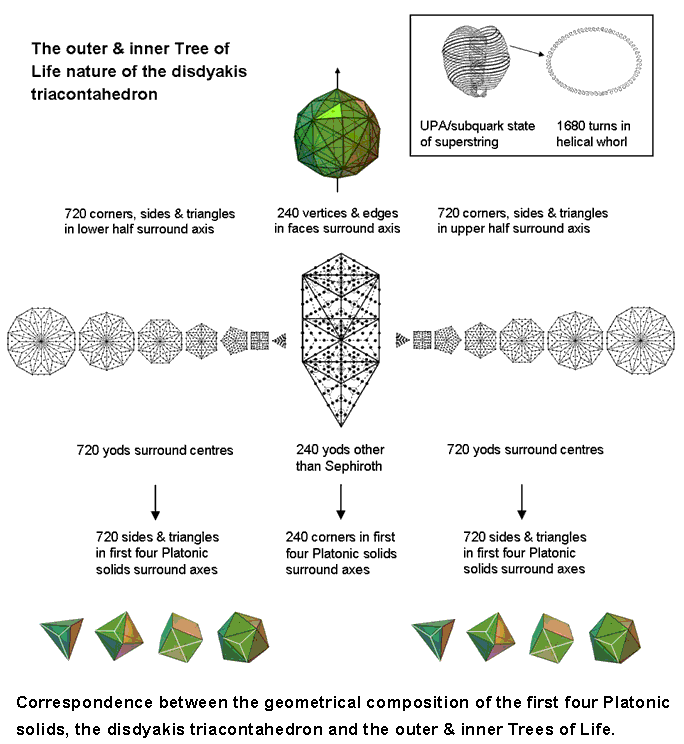Figure 7.

Outer & inner forms of the 1-tree

When its 19 triangles are Type A, the 1-tree contains 240 yods other than their 11 corners. Each set of the seven separate Type B polygons making up its inner form has 720 yods surrounding their centres.

1st 4 Platonic solids

When the F faces of a Platonic solid with E edges are each divided into their m sectors and their vertices & face centres are joined to the centre of the polyhedron, creating internal triangles that are treated as Type A, the number of corners, sides & triangles that surround an axis passing through its centre and two opposite vertices = 10E + 9mF. The tetrahedron* (E = 6; mF = 12) has 168 such geometrical elements, both the octahedron (E = 12; mF = 24) & cube (E = 12; mF = 24) have 336 elements and the icosahedron (E = 30; mF = 60) has 840 elements. The axes of the first four Platonic solids, which the ancient Greeks believed were the shapes of the particles of the elements of Fire, Water, Earth & Air, are surrounded by 1680 geometrical elements (840 in the tetrahedron, octahedron & cube and 840 in the icosahedron). They comprise 240 corners and 720 sides & triangles in each set of their halves.

* In the case of the tetrahedron, pairs of whose vertices are not diametrically opposite each, any axis that passes through its centre and any two vertices is not straight. Despite this, its external and internal geometrical elements can be divided into two similar sets associated with two fictitious "halves" because it has an even number of vertices, an even number of edges & an even number of faces.

Disdyakis triacontahedron

When the 62 vertices of the disdyakis triacontahedron with 180 edges are joined to its centre and the resulting 180 internal triangles turned into Type A triangles, 1680 corners, sides & triangles in its faces and interior surround an axis passing through any two diametrically opposite vertices. They comprise (60+180=240) vertices & edges and 1440 corners (720 in each half of the polyhedron).

In conclusion, the fact that a subset of the geometrical elements in the 120-cell has a division: 1680 = 720 + 240 + 720 that manifests in at least three other sacred geometries is further evidence refuting the possibility that it is just by chance that this polychoron embodies the number 16800.

3) There are 2640 (=264×10) vertices, edges, pentagonal faces & dodecahedral cells, where 264 is the number of yods in the seven enfolded Type A polygons (see Figure 5).

4)  It has 1320 (=132×10) yods at corners of 3600 tetractyses in its 720 pentagonal faces. 132 is the 66th even integer, where 66 is the 65th integer after 1 and 65 is the number value of ADONAI, the Godname of Malkuth.

5)

The 120-cell

600-cell

1) The 360 sectors of its 1200 triangular faces contain 1320 (=11×120) corners, of which 120 are vertices, leaving 1200 (=10×120) corners, where 1200 = (1+2+3+4)×(22+42+62+82) = 10×sum of the first 10 odd integers (see (2).

2) Surrounding the centre of the 600-cell are 14400 (=1202) geometrical elements, where

 3 5 7 120 = 7 9 11 13 15 17 19

Is the sum of the first 10 odd integers after 1. This beautiful property shows how the Decad determines the geometrical composition of the 600-cell. As 120 = 5!, it is also determined by the Tetrad because 14400 is the square of the factorial of the fourth integer after 1. The number of points surrounding its centre = 2040 = 24×85 = 1×2×3×4(40+41+42+43), showing that it, too, is expressed by the Tetrad. Of these, 120 (= 23 + 24 + 25 + 26) are vertices, leaving 1920 points, where 1920 = 64×30 = 26(21+22+23+24) = 27 + 28 + 29 + 210. The number of triangles = 5760 = 10×242 = (1+2+3+4)×12×22×32×42. The number of triangles in its 600 faces = 3600 = 4×900 = 4×302 = 4×(12+22+32+42)2. The 9240 points, lines & triangles in its faces include (120+720=840) vertices & edges and 8400 new geometrical elements created by its construction from Type A triangles. This is easily seen from the fact that inside each of the 1200 faces as Type A triangles are a corner & three sides of three triangles, i.e., seven geometrical elements, so that (1200×7=8400) new elements are generated when the faces become Type A triangles: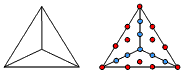Inside the Type A triangle are one corner, 3 sides & 3 triangles, i.e., 7 geometrical elements. 7 internal blue yods line sides of tetractyses.

840 = 84×10 = (12+32+52+72)×(1+2+3+4) and 8400 = (12+32+52+72)×(13+23+33+43). As 120 =5! and 720 = 6!, 840 = 5! + 6!. As each edge is the side of an internal Type A triangle, the 5160 internal geometrical elements surrounding the centre comprise 5! sides terminating on vertices and (7×720=7!) geometrical elements generated by the edges, i.e., 5160 = 5! + 7!.

The possession by the 600-cell of 840 vertices & edges and 10 times as many more geometrical elements in its faces when they are Type A polygons is an unambiguous indication of the relevance of this polychoron to superstring theory. This is because both the number 840 and the number 8400 are superstring structural parameters, being, respectively, the number of turns in an outer or inner half of, respectively, a single, helical whorl and all 10 whorls of the UPA examined with micro-psi by Annie Besant & C.W. Leadbeater. The 120-cell embodies the superstring 16800, but only as a subset of its geometrical elements, namely, the 16800 elements surrounding its centre other than centres of Type A faces and Type A internal triangles. Is, however, the display of superstring structural parameters like 168, 840, 1680, etc unique to the 600‑cell? The number of geometrical elements other than vertices and edges needed to divide each of the F faces of a polychoron into m sectors is (2m+1)F. Table 5 shows this number for the six polychorons. Also displayed is the number (C+S) of the corners & sides of the triangles in their faces.

Table 5. Number of geometrical elements other than vertices & edges generating Type A faces.

 Polychoron Vertices V Edges E Faces F V+E m (2m+1)F C+S 5-cell 5 10 10 15 3 70 70 8-cell 16 32 24 48 4 216 168 16-cell 8 24 32 32 3 224 160 24-cell 24 96 96 120 3 672 504 120-cell 600 1200 720 1800 5 7920 6120 600-cell 120 720 1200 840 3 8400 5640

We see that the numbers 840 and 8400 appear only in the 600-cell. However, the 24 faces of the 8-cube have 168 points & lines in addition to its 48 vertices & edges. Notice from Table 2 that it also appears in the 16-cell (the dual of the 8-cell) as the number of triangles in its 32 faces and interior. Discounting coincidence, this appearance of the number 168 suggests that both polytopes play a role in the structure of the UPA/superstring that is yet to be discovered (see #4 for more details). That the tesseract conforms to the archetypal pattern represented by the I Ching table of 64 hexagrams is proved here.

En passim, let us consider two 600-cells sharing the same centre, one a smaller version of the other. Surrounding their centre are (1320+1320=2640=264×10) points in their 2400 faces. They consist of 240 (=24×10) vertices and 2400 (=240×10) new points generated by dividing their faces into their sectors. Compare this with the fact that the seven enfolded polygons of the inner Tree of Life contain 264 yods, of which 24 lie outside the root edge as corners of the first six enfolded polygons (a subset of the set of seven polygons that is holistic in itself), leaving 240 yods. It is evidence that the two concentric 600-cells conform to the inner form of the Tree of Life. The significance of this for superstring physics will be revealed on the next page. Surrounding their shared centre, the two 600-cells have 240 vertices and 3840 other points in their faces and interiors, where 240 = 24 + 25 + 26 + 27 and 3840 = 28 + 29 + 210 + 211. The reason for considering two 600-cells will be explained on the next page.

3) There are 2640 (=264×10) vertices, edges, triangular faces & tetrahedral cells. The 600-cell embodies the Tree of Life parameter 264 (see Figure 5). It is yet more evidence for this polychoron being the polytope counterpart of either half of the inner Tree of Life.

4) The number of yods in its 1200 faces is 13560, that is, the number of yods in 1356 tetractyses. The number 1356 is the number of yods surrounding the centres of the 14 enfolded Type B polygons, which contain 1370 yods (see Fig. 6). 13560 yods surround the (70+70=140) centres of the (70+70) polygons enfolded in 10 separate Trees of Life. This is remarkable evidence for the holistic character of the 600-cell, for in each case this number determines the form of the two equivalent geometries. The inner Tree of Life shares with its outer form seven yods on the vertical diameter of each hexagon, one of which is the centre of this polygon, as well as the centre of each triangle, which coincides with a hexagonal yod on the Chesed-Geburah Path of the outer Tree of Life. Each set of seven polygons shares six yods that are not centres of polygons. The number of these shared yods in the (7+7)n polygons enfolded in the n-tree = 5n + 1 + 5n +1 = 10n + 2. The number of yods in these polygons = 1368n + 2. The number of yods surrounding their centres = 1368n + 2 – 7n – 7n = 1354n + 2. The number of such yods that are intrinsic to the polygons in the sense that they are unshared with the n-tree = 1354n + 2 – (10n+2) = 1344n. The number of yods surrounding their centres that are intrinsic to the (7+7) polygons enfolded in successive Trees of Life = 1344. As 336 = 22 + 62 + 102 + 142, 1344 = 4×336 = 22(22+62+102+142) =  42 + 122 + 202 + 282, i.e.,  the number of yods surrounding centres of polygons that are intrinsic to the inner form of successive Trees is the sum of the squares of four integers separated by eight units, the first being 4, which is the Tetrad. Four of these yods lie on the root edge shared by the two sets of polygons, leaving 670 (=67×10) yods outside the root edge surrounding centres of polygons that are intrinsic to each set of seven enfolded polygons. The number value 67 of Binah determines how many yods surrounding centres of polygons are intrinsic to the inner form of successive Trees. This clearly illustrates the formative character of the archetype embodied in this Sephirah.

The (70+70) polygons enfolded in the 10-tree have 13440 intrinsic yods surrounding their 140 centres. This is the number of yods other than vertices in the faces of the 600-cell: 13560 – 120 = 13440. Given the 10-tree and the 140 centres of the polygons making up its inner form, 13440 more yods are needed to construct the latter from Type B triangles. In an analogous way, given the 120 vertices of the 600-cell, 13440 more yods are needed to construct it from tetractyses. Discounting the possibility of coincidence as implausible, it strongly suggests that the 600-cell is the 4‑dimensional polychoron counterpart of the inner form of 10 overlapping Trees of Life. The archetypal nature of the latter has been analysed in depth in many previous articles, as has its equivalence to other sacred geometries, such as the disdyakis dodecahedron. Moreover, the analogy is unique, for the faces of the 120-cell (the only other polychoron whose yod population is an integer multiple of 10) have 14520 yods — more than the 13682 yods in the (70+70) polygons enfolded in the 10-tree.

5) The number of yods lining the 720 edges connecting its 120 vertices = 120 + 2×720 = 1560 = 156×10, where 156 is the 155th integer after 1. ADONAI MELEKH, the Godname of Malkuth with number value 155, appropriately prescribes the form of the 600-cell.

6) 21000 (=21×1000) yods surround its centre. This is a remarkable example of how Godnames prescribe objects with holistic character (as we shall see shortly), for 21 is the number value of Kether, the first Sephirah.

7) The 600-cell is composed of 600 tetrahedra, joined five to an edge.

8) 9960 yods line the 3600 tetractyses in its faces. As found in (5), 1560 yods line the 720 edges. This leaves (9960−1560=8400) more boundary yods (seven inside each face) needed to construct its 1200 faces from Type A triangles. This is the same as the number of new geometrical elements needed for their construction, as found in (1). Once again, the superstring structural parameter 8400 appears as the number of yods lining the 3600 tetractyses other than those on its edges.

One of the many remarkable properties of the 600-cell is that, if its edge is one unit long, its circumradius (centre-vertex distance) is the Golden Ratio Φ = (1+√5)/2. It is unique amongst the six polychorons in having this beautiful property (see http://davidf.faricy.net/polyhedra/Polytopes.html). Both the 16-cell and the 24-cell with unit edge lengths have a circumradius of 1.

The 600-cell

 << Previous      2  3  4  5  ...24    Next >>

Home